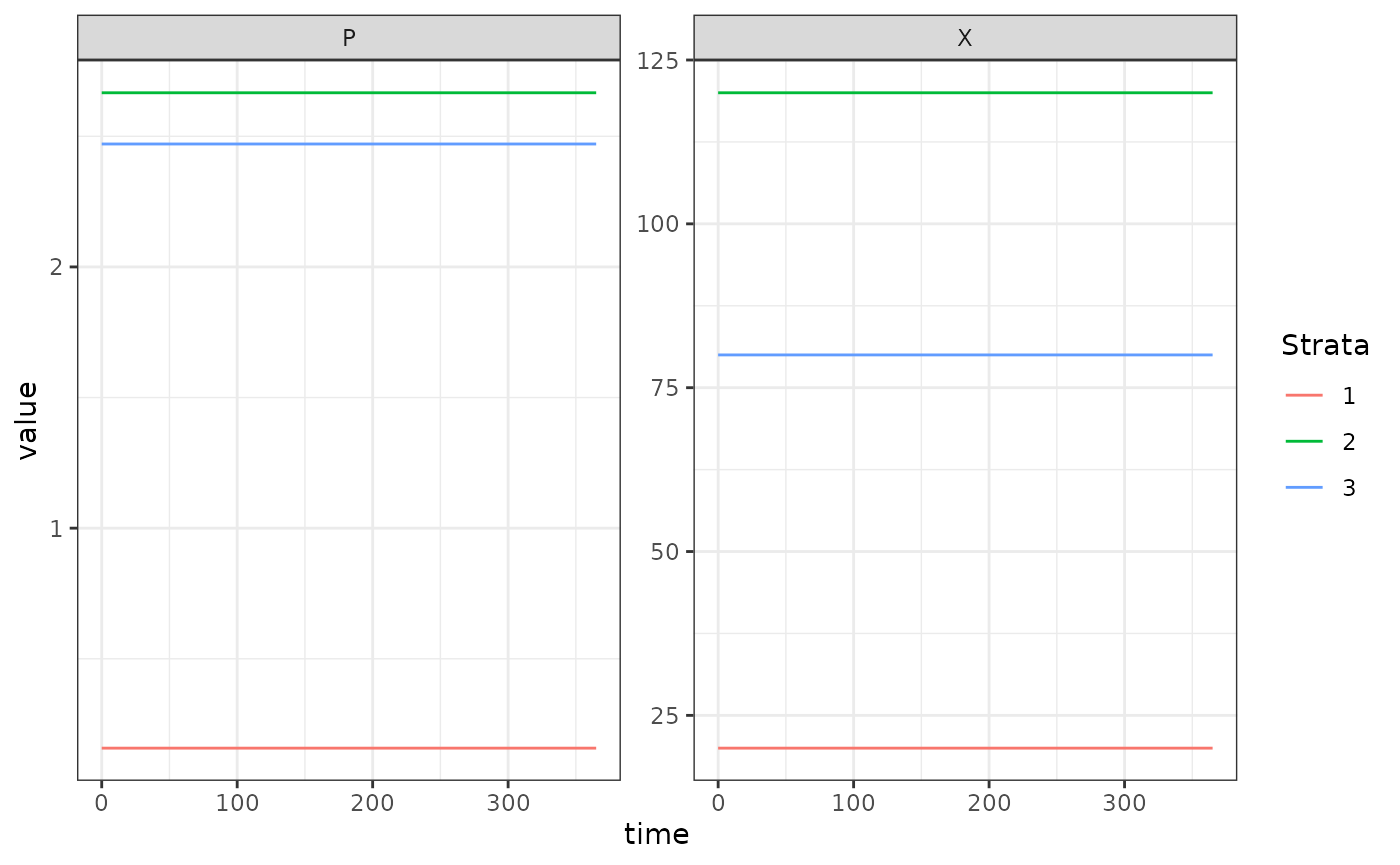The basic SIP (Susceptible-Infected-Prophylaxis) human model model fulfills the generic interface of the human population component. It is a reasonable first complication of the SIS human model. This requires two new parameters, $$\rho$$, the probability a new infection is treated, and $$\eta$$ the duration of chemoprophylaxis following treatment. $$X$$ remains a column vector giving the number of infectious individuals in each strata, and $$P$$ the number of treated and protected individuals.

## Differential Equations

The equations are as follows:

$\dot{X} = \mbox{diag}((1-\rho)bEIR)\cdot (H-X-P) - rX$

$\dot{P} = \mbox{diag}(\rho b EIR) \cdot (H-X-P) - \eta P$

## Equilibrium solutions

Again, we assume $$H$$ and $$X$$ to be known. and solve for $$EIR$$ and $$P$$.

$P = \mbox{diag}(1/\eta) \cdot \mbox{diag}(\rho/(1-\rho)) \cdot rX$

$EIR = \mbox{diag}(1/b) \cdot \mbox{diag}(1/(1-\rho)) \cdot \left( \frac{rX}{H-X-P} \right)$

Given $$EIR$$ we can solve for the mosquito population which would have given rise to those equilibrium values.

## Example

library(exDE)
library(deSolve)
library(data.table)
library(ggplot2)

Here we run a simple example with 3 population strata at equilibrium. We use exDE::make_parameters_X_SIP to set up parameters. Please note that this only runs the human population component and that most users should read our fully worked example to run a full simulation.

We use the null (constant) model of human demography ($$H$$ constant for all time).

nStrata <- 3
H <- c(100, 500, 250)
membershipH <- rep(1,3)
searchWtsH <- rep(1,3)
X <- c(20, 120, 80)
b <- 0.55
c <- 0.15
r <- 1/200
eta <- c(1/30, 1/40, 1/35)
rho <- c(0.05, 0.1, 0.15)
Psi <- matrix(data = 1,nrow = 1, ncol = nStrata)

P <- diag(1/eta) %*% diag(rho/(1-rho)) %*% (r*X)
EIR <- diag(1/b, nStrata) %*% diag(1/(1-rho)) %*% ((r*X)/(H-X-P))

params <- make_parameters_xde()
params$nStrata = nStrata params$nPatches = 1

params = make_parameters_demography_null(pars = params, H=H, membershipH=membershipH, searchWtsH=searchWtsH, TimeSpent=Psi)

params = make_parameters_X_SIP(pars = params, b = b, c = c, r = r, rho = rho, eta = eta)
params = make_inits_X_SIP(pars = params, X, as.vector(P))
params = make_indices(params)

y0 <- get_inits_X(params)

dXdt_local = function(t, y, pars, EIR) {
list(dXdt(t, y, pars, EIR))
}

out <- deSolve::ode(y = y0, times = c(0, 365), dXdt_local, parms = params, method = 'lsoda', EIR = as.vector(EIR))

colnames(out)[params$X_ix+1] <- paste0('X_', 1:params$nStrata)
colnames(out)[params$P_ix+1] <- paste0('P_', 1:params$nStrata)

out <- as.data.table(out)
out <- melt(out, id.vars = 'time')
out[, c("Component", "Strata") := tstrsplit(variable, '_', fixed = TRUE)]
out[, variable := NULL]

ggplot(data = out, mapping = aes(x = time, y = value, color = Strata)) +
geom_line() +
facet_wrap(. ~ Component, scales = 'free') +
theme_bw()